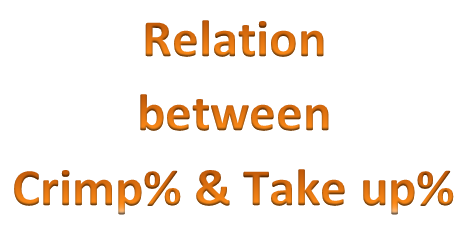# Textile Calculation

Problems and solutions of textile

## Relation between Crimp% and Take up %

Relation between Crimp% and Take up %
Raju Tex
Knitting Technologist,
B.J.Group, Mawna, Gazipur
Chittagong Textile Engineering College

Crimp Percentage %:
Crimp Percentage is defined as the mean difference between the straightened thread length and the distance between the ends of the thread while in cloth, expressed as a percentage.

L-p
Crimp%, C =…… X 100     ……………………….(1)
p

Straightened length – crimped length
= …………………………………………
Crimped length

Take up percentage:
Take up percentage or crimp rigidity is a measure of the ability of textured yarn to receive from stretch and is related to the bulking properties of the yarn before weaving and the length of yarn in the fabric after weaving, expressed as a percentage of the length of yarn before weaving, is called take up percentage.

L-p
Take up percentage, T = …….. X 100 ……………………… (2)
L

Where,
T = Take up percentage
L = Length of yarn before weaving
p = Length of yarn in fabric after weaving

Relation between Crimp% and Take up %:From equation (1)

L-p
C = …….. X 100
p

=> C/100 = L/p – 1

=> L/p = C/100 + 1

100 + C
=> L/p = …………        …………………(3)
100

From equation (2)

L-p
T = ……….. X 100
L

= (1- p/L) X 100

= {1 – 100/(100+C)} X 100 ……………………….. [from equation 3]

100C
So, T = …………     ……………………………(4)
100+C

To express percentage crimp in terms of take up %, equation (4) is solved as follows:

100C
T = ……………
100+C

=> 100C = 100T + CT

=> 100C – CT = 100T

100T
=> C = …………….        ……………………..(5)
100 – T

Equation (4) and (5) are two relations between Crimp% and Take up%